# GED Math : Area of a Triangle

## Example Questions

1 3 Next →

### Example Question #21 : Area Of A Triangle

Determine the area of a triangle with a base of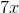and a height of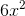.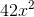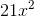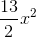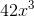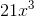Explanation:

Write the formula for the area of a triangle.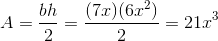The answer is:### Example Question #22 : Area Of A Triangle

Find the area of a triangle with a base of 9in and a height that is two times the base.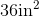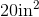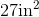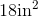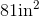Explanation:

To find the area of a triangle, we will use the following formula: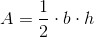where b is the base and h is the height of the triangle.

Now, we know the base of the triangle is 9in. We know the height is two times the base. Therefore, the height is 18in. So, we can substitute. We get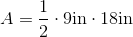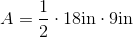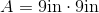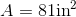### Example Question #23 : Area Of A Triangle

Find the area of a triangle with a height of 40 and a base of 20.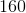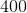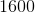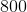Explanation:

Write the formula for the area of a triangle.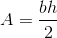Substitute the base and height into the equation.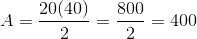The answer is:### Example Question #24 : Area Of A Triangle

Find the area of a triangle with a base of 6 and a height of 30.Explanation:

Write the formula for the area of a triangle.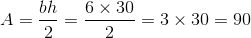The answer is:### Example Question #25 : Area Of A Triangle

What is the area of a triangle with a base of 8 and a height of 30?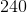Explanation:

Write the formula for the area of a triangle.Substitute the dimensions.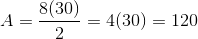The answer is:### Example Question #26 : Area Of A Triangle

Find the area of a triangle with a base of 100 and a height of 300.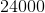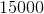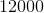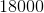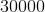Explanation:

Write the formula for the area of a triangle.Substitute the base and the height into the equation.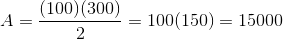The answer is:### Example Question #27 : Area Of A Triangle

You want to build a garden in the shape of a right triangle. If the two arms will be 6ft and 8ft, how much area will the garden take up?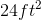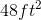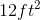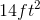Explanation:

You want to build a garden in the shape of a right triangle. If the two arms will be 6ft and 8ft, how much area will the garden take up?

To find the area of a triangle, use the following formula.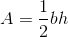Note that in a right triangle, our two arms correspond to our base and our height. Furthermore, it does not matter which is which, because when we multiply, order does not matter.

So, to find our area, simply plug in and simplify.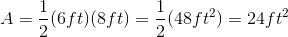So, our answer is 24 ft squared

### Example Question #28 : Area Of A Triangle

What is the area of an equilateral triangle that has a perimeter of? Round your answer to the nearest hundredths place.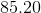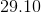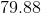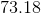Explanation:

Start by finding the length of a side of the triangle.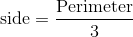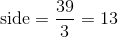Next, recall how to find the area of an equilateral triangle.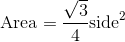Plug in the length of the side to find the area.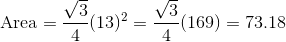### Example Question #29 : Area Of A Triangle

If the height of a triangle is twice the length of the base, and the base length is 3.5 inches, what is the area of the triangle?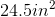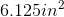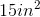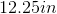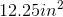Explanation:

First we need to know that the formula for area of a triangle: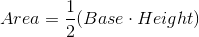We know that our base is 3.5 inches, and our height is twice that, which is 7 in.

Now we can plug in our base and height to the equation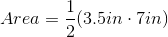Multiply and solve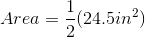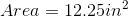### Example Question #30 : Area Of A Triangle

What is the area of a triangle with a height that is three times the length of the base, if the base is 4cm?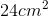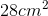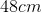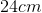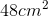Explanation:

First we need to recall the formula for area of a triangle: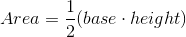We know that our base is 4cm, and our height is 3 times the length of the base, since 4x3=12 we know that our height is 12cm

Now we can plug in our numbers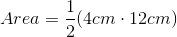First we multiply 4 and 12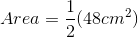Next we distribute the fraction which is the same as dividing in half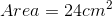Notice our answer is in centimeters since we multiplied two terms measured in centimeters

1 3 Next →

### All GED Math Resources